Read Mode
Default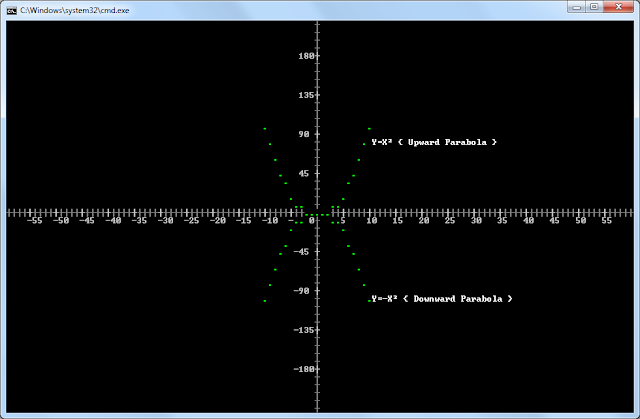Plot Function ver.2.0 by Kvc
Hello Batchers, We’re back with an upgrade to the older version of Plot function. The plot function ver.1.0 is really an amazing function; it is based on pure mathematics. As you know that, it can plot various points (co-ordinates) on the console, in the same manner as we are plotting in our math’s class. Graphs help us in many ways; they provide simple and easy view of a complex equations or problems. You can analyze any system using the graphs or plots. Although there are many advanced computer languages, which can easily implement this requirement.

But here, we’re trying to understand “how they became so much advance??” using our batch programming skills. Playing with mathematics is such a FUN. When you try to calculate and achieve something and only one mathematics technique can provide you everything. The feeling of getting the thing we want by our own understood methods is amazing. Now, without wasting any time… Let’s talk about this version of plot function.

In previous version, we’ve achieved the displacement of CMD’s default origin from TOP-LEFT CORNER to the center of the console window, with the ability to plot co-ordinates according to the shifted NEW-ORIGIN. But, the problem with Ver.1.0 was that, it can only plot on console according to the size of the console. I.e. you can’t plot 45, 45 on the console screen with size of 25x80, this is because 45 of Y lie outside the console co-ordinate limit, as console has 25 lines. Thus it gives different output than original.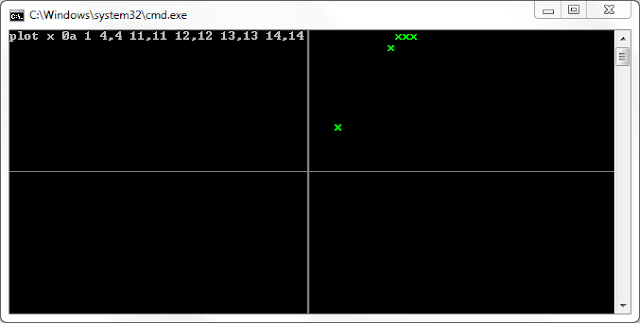Problem with ver.1.0
As 13 is above than the limit of Y-Line (‘cuz 12 above and 12 below, 1 at center, total 25 lines), so all other Co-ordinates above than the console size limit will be place somewhere else. To show these required co-ordinates, you need to change the size of the console window (via ‘Mode’ command) and then re-plot these co-ordinates. But it is not possible to change the console screen size every time. The function should be intelligent enough to understand the points and adjust itself according to that.

So, for fulfilling this requirement, we analyzed the function more and tried to make some more calculations to adjust the output according to the input given by the user. After spending some hours with pen and copy, we finally made mathematical modeling of the requirement we want in the function. Now, the function started working a little intelligently than previous version.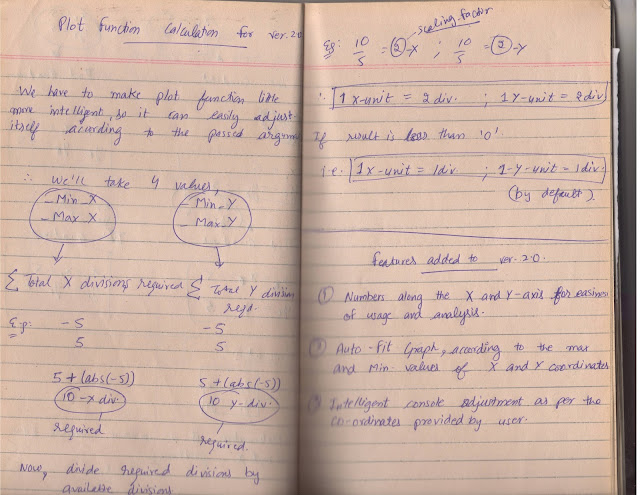Our work-book
But the problem was not solved completely yet. The user knows the position of the plots as he has given the input. But there is no method of determining the value of a point by simply looking at the graph. Now, the algorithm for displaying the numbers besides the x-axis and y-axis should be built. After few hours and with lot of efforts, we finally did it.Advancement in version 1.0
Now, you can even print and analyze some of the mathematical equations of various shapes. As we have tested parabola and line equations. The same above program gives the following output with the version 1.0 of this plot function.Problem with ver.1.0
And now, we know that, by watching both images…you’ll know the difference and upgrade of ver.2.0. All the usage and file-input to the function is same as of ver.1.0. But still for the formality purpose, we have to show the usage and input file example to the users, before they download and start using this awesome batch extension.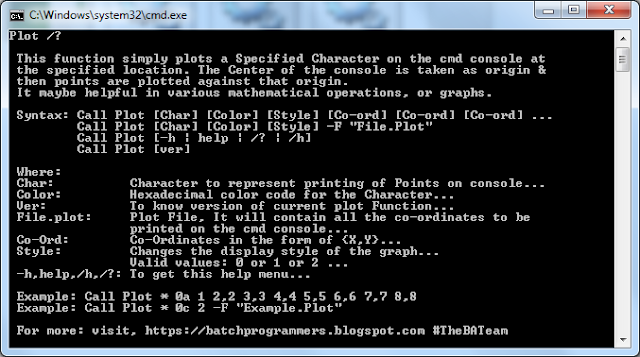Help Menu
And the layout or format of the plot file is same as of v.1.0. All lines starting with ‘#’ are comments and rest you can see in the image or download and analyze the properties of the Plot file by your own.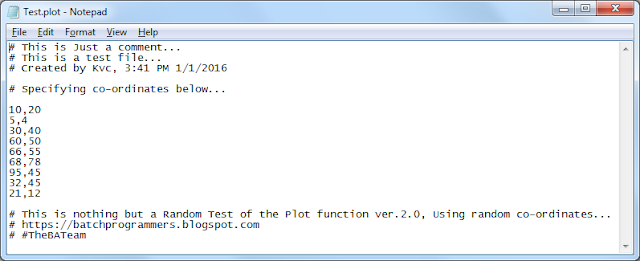Plot File "Example"
You can even find the intersection of two equations graphically via using this plot function. For example, to find the intersection of Lines ‘Y=5x+5’ and ‘Y=-5x+5’, just calculate the corresponding values of ‘Y’ with respect to ‘X’ using a for loop, for both the equations and plot the co-ordinates using this plot function.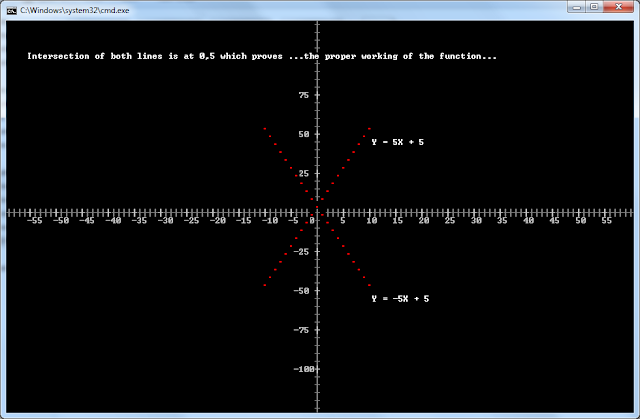An Example of Usage
As you can see in the image above that, the intersection is at 0,5 position. You can verify it by solving both the equations mathematically also. At last, we hope you’ll like our work. Give your response and suggestions via your comments below.

Keep learning, keep sharing...
Be Happy, Spread Happiness...

#TheBATeam